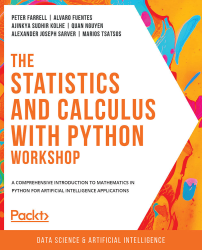•#### The Statistics and Calculus with Python Workshop#### Overview of this book

Are you looking to start developing artificial intelligence applications? Do you need a refresher on key mathematical concepts? Full of engaging practical exercises, The Statistics and Calculus with Python Workshop will show you how to apply your understanding of advanced mathematics in the context of Python. The book begins by giving you a high-level overview of the libraries you'll use while performing statistics with Python. As you progress, you'll perform various mathematical tasks using the Python programming language, such as solving algebraic functions with Python starting with basic functions, and then working through transformations and solving equations. Later chapters in the book will cover statistics and calculus concepts and how to use them to solve problems and gain useful insights. Finally, you'll study differential equations with an emphasis on numerical methods and learn about algorithms that directly calculate values of functions. By the end of this book, you’ll have learned how to apply essential statistics and calculus concepts to develop robust Python applications that solve business challenges.
Preface1. Fundamentals of PythonFree Chapter
2. Python's Main Tools for Statistics3. Python's Statistical Toolbox4. Functions and Algebra with Python5. More Mathematics with Python6. Matrices and Markov Chains with Python7. Doing Basic Statistics with Python8. Foundational Probability Concepts and Their Applications9. Intermediate Statistics with Python10. Foundational Calculus with Python11. More Calculus with Python12. Intermediate Calculus with PythonAppendix# An Overview of Statistics

In this section, we will briefly discuss the goal of the overarching field of statistics and talk about some of its fundamental ideas. This conversation will set the context for the subsequent topics in this chapter and this book.

Generally speaking, statistics is all about working with data, be it processing, analyzing, or drawing a conclusion from the data we have. In the context of a given dataset, statistics has two main goals: describing the data, and drawing conclusions from it. These goals coincide with the two main categories of statistics — descriptive statistics and inferential statistics — respectively.

In descriptive statistics, questions are asked about the general characteristics of a dataset: What is the average amount? What is the difference between the maximum and the minimum? What value appears the most? And so forth. The answers to these questions help us get an idea of what the dataset in question constitutes and what...